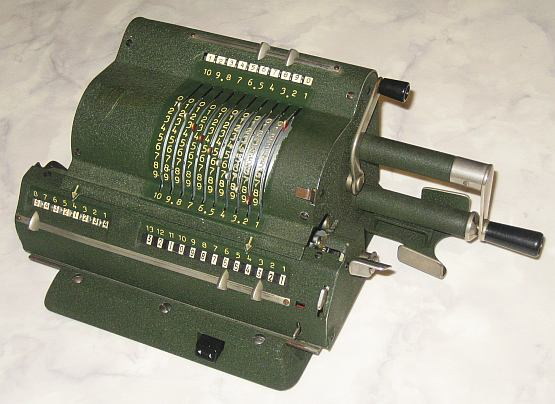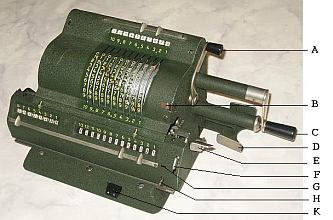previous <<==>> next``` Have a look at the Restoration Procedure ... TECHNICAL DATA of the Mechanical Calculator ADDO » MULTO-13 « ************************************************************************** Serial Number: 13-12363 Dimensions: (ca.) Width = 11 " / 28 cm Depth = 6_1/2" / 17 cm Height = 5_1/4" / 13 cm Weight: (ca.) 12_1/3 lbs / 5.6 kg Mechanics: Pin-Wheel / Sprossenrad 10s Carry Mechanism Counter Stepping Mechanism + Animation Functions: Add, Subtract, Multiply, Divide Back-Transfer / Rueckuebertragung Registers: Input = 10 Decimals Counter = 8 Decimals (NO Carry; Neg.Figures RED) Arithmetic = 13 Decimals Manufacturer: A.B. ADDO Malmo Sweden 1955 H O W T O U S E the ADDO » MULTO-13 « ********************************************* BASIC SETTINGS: ===============(a) Crank: ---------- For addition (+) the crank [C] is turned clockwise; for subtraction (-) the crank is turned counter-clockwise. In idle position the crank is down & locked. To make one or more turns with the crank, pull out the handle to unlock. When finished the turn(s), let the handle snap into the lock again. The locked down position only makes other functions accessible. REMARK: Every started turn has to be finished completely! Accidentally started turns are correctible somehow. (b) Clearing Registers: ----------------------- I N P U T : Set by 10 levers, the content is shown also in the display. In operation the levers and its displays (!) rotate. To clear the input regster pull the lever [A] over the crank. A R I T H M E T I C & C O U N T E R : Pull the big lever [E] right on the carriage. C O U N T E R O N L Y : (1st) pull the ( vertical, small ) silver lever [F] right in front of the carriage and (2nd) pull the big lever [E] right on the carriage. (c) Shifting the Carriage: -------------------------- Push or pull the twin-lever [D] under the crank and the carriage will move one step left or right. When the black button [K] right in front of the base is pressed, the carriage jumps left in position "1" by force of a spring. Position "1" is the "Start Position". The carriage can be shifted quick to the right by hand. (d) Back Transfer: ------------------ For continuous multiplication the contents of the arithmetic unit can be back transfered into the input register with the following procedure: Pull the input-clear lever [A] & HOLD it. Push the SMALL LEVER [B] right of the input unit. RELEASE the input-clear lever [A]. Now on front of the carriage a BACK-TRANSFER INDICATOR shows (1st) in the WINDOW [G] a RED FLAG and (2nd) the LEVER (H) under it is LIFTED. Pull the arithmetic clear-lever [E]. The former content of the arithmetic register is now transfered back into the input register and has set its levers and displays accordingly. ===>> R E M A R K : The back-transfer can be interrupted by pushing down the LIFTED LEVER [H]. ADDITION & SUBTRACTION: ======================= Example: 123 + 45 - 6 = 162 Clear input, counter and arithmetic units. ADD: Enter the first number (123) in the far right of the input unit. Make a positive (clockwise) turn with the crank to transfer the number into arithmetic unit. The counting unit displays the figure 1. Enter the second number (45). Make a positive (clockwise) turn with the crank to add the number. The arithmetic unit displays the intermediate sum (168) and the counting unit displays the figure 2. SUBTRACT: Enter the third number (6). Make a negative (counter-clockwise) turn with the crank. The arithmetic unit displays the result (162) and the counting unit is decreased by 1. REMARK: NEGATIVE RESULTS are displayed in the arithmetic unit as the COMPLEMENT of the next higher 10, 100, 1000, ... Example: -12 = 99...9988 MULTIPLICATION: =============== Example: 123 x 45 = 5535 Clear input, counter and arithmetic units. Enter the multiplicand (123) in the far right of the input unit. The multiplicator (45) has two digits, so the carriage is shifted to position 2. Make positive (clockwise) turns with the crank, until the first figure of the multiplicator (4) will appear in the 2nd position of the counter unit. Shift the carriage to position 1. Repeat making positive turns with the crank, until the second figure of the multiplicator (5) appears in the 1st position of the counter unit. The multiplication is done: The multiplicand (123) stays in the input unit, the multiplicator (45) in the counter and the result (5535) is in the arithmetic unit. DIVISION: ========= Example: 22 : 7 = 3.1428571 Remainder 3 Division requires 3 steps: (1st) To Set the Dividend into Arithmetic Unit: ----------------------------------------------- For the maximum number of decimals, pull out the carriage to the far right position. Enter the dividend (22) in the far right of the input unit. Make a positive (clockwise) turn with the crank to transfer into arithmetic unit. (2nd) To Set the Divisor into Input Unit: ----------------------------------------- Clear the counter. Enter the divisor (7) above the dividend (22). (3rd) To Divide: ---------------- Make negative (counter-clockwise) turns with the crank until the arithmetic unit shows an "underflow". Make one positive (clockwise) turn with the crank. Move the carriage to the next left position. Repeat this procedure until the required number of decimals ... The result (3.1428571) is in the counter unit ( in RED ), and the remainder (3) is in the arithmetic unit. The divisor (7) stays in the input unit, therefore an additional decimal can be estimated ... ( 4, cause 4 x 7 = 28). R E M A R K : ------------- The COUNTER-FIGURES ( WHITE/RED ) follows the "ODHNER-TYPE"-Scheme Have a look at "Calculating Trickies" ... Have a look at the Restoration Procedure ... impressum: ************************************************************************** © C.HAMANN http://public.BHT-Berlin.de/hamann 03/21/12 ```